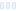Tangerine Hydration Multiplier

Liquid I.V.

Main info:

Tangerine Hydration Multiplier
Liquid I.V.
1 stick
45 Calories
11 g
0 g
0 g

0 g
0 mg
0 g
500 mg
11 g
0 g

Percent calories from...
Nutrition Facts
For a Serving Size of (g)
How many calories are in Tangerine Hydration Multiplier? Amount of calories in Tangerine Hydration Multiplier: Calories Calories from Fat (%)
% Daily Value *
How much fat is in Tangerine Hydration Multiplier? Amount of fat in Tangerine Hydration Multiplier: Total Fat
How much sodium is in Tangerine Hydration Multiplier? Amount of sodium in Tangerine Hydration Multiplier: Sodium
How much potassium is in Tangerine Hydration Multiplier? Amount of potassium in Tangerine Hydration Multiplier: Potassium
How many carbs are in Tangerine Hydration Multiplier? Amount of carbs in Tangerine Hydration Multiplier: Carbohydrates
How many net carbs are in Tangerine Hydration Multiplier? Amount of net carbs in Tangerine Hydration Multiplier: Net carbs
How much sugar is in Tangerine Hydration Multiplier? Amount of sugar in Tangerine Hydration Multiplier: Sugar
How much fiber is in Tangerine Hydration Multiplier? Amount of fiber in Tangerine Hydration Multiplier: Fiber
How much protein is in Tangerine Hydration Multiplier? Amount of protein in Tangerine Hydration Multiplier: Protein
Vitamins and minerals
How much Calcium is in Tangerine Hydration Multiplier? Amount of Calcium in Tangerine Hydration Multiplier: Calcium
How much Iron is in Tangerine Hydration Multiplier? Amount of Iron in Tangerine Hydration Multiplier: Iron
Fatty acids
Amino acids
* The Percent Daily Values are based on a 2,000 calorie diet, so your values may change depending on your calorie needs.Loading similar foods...
Note: Any items purchased after clicking our Amazon buttons will give us a little referral bonus. If you do click them, thank you!Be cool

- Zen orangeI never skip arm day

- Buff broccoli